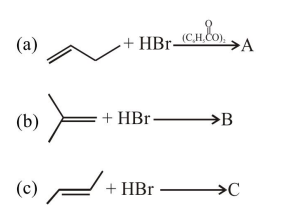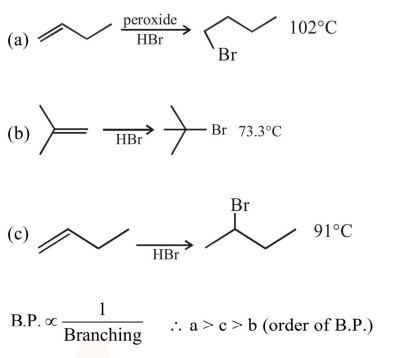# The increasing order of the boiling points of the major products A,

Question:

The increasing order of the boiling points of the major products A, B and C of the following reactions will be :1. $\mathrm{C}<\mathrm{A}<\mathrm{B}$

2.  $\mathrm{B}<\mathrm{C}<\mathrm{A}$

3. $\mathrm{A}<\mathrm{B}<\mathrm{C}$

4. $\mathrm{A}<\mathrm{C}<\mathrm{B}$

Correct Option: , 2

Solution: